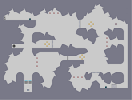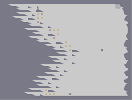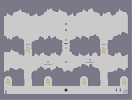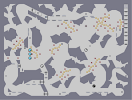Lugia V1Hover over the thumbnail for a full-size version.

Author Riobe author:riobe fun-to-look-at lugia n-art nonplayable pokenart rated 2008-08-21 2008-08-21 4 by 13 people. \$Lugia V1#Riobe#none#00000000000000000000000000000000000000000000000000000000000000000000000000000000000000000000000000000000000000000000000000000000000000000000000000000000000000000000000000000000000000000000000000000000000000000000000000000000000000000000000000000000000000000000000000000000000000000000000000000000000000000000000000000000000000000000000000000000000000000000000000000000000000000000000000000000000000000000000000000000000000000000000000000000000000000000000000000000000000000000000000000000000000000000000000000000000000000000000000000000000000000000000000000000000000000000000000000000000000000000000000000000000000000000000000000000000000000000000000000000000000000000000000000000000000000000000000000000000000000|10^530,576!10^532,575!10^533,575!10^539,572!10^542,571!10^546,570!10^550,568!10^552,566!10^555,564!10^559,560!10^560,559!10^567,556!10^569,555!10^571,555!10^498,565!10^500,564!10^503,562!10^507,560!10^511,558!10^513,556!10^514,554!10^518,553!10^518,553!10^517,552!10^512,552!10^509,552!10^509,552!10^507,552!10^500,553!10^499,553!10^496,552!10^496,552!10^494,552!10^492,552!10^490,552!10^488,551!10^488,551!10^488,551!10^490,551!10^493,547!10^496,546!10^499,544!10^500,544!10^512,536!10^518,533!10^520,531!10^523,530!10^523,530!10^524,530!10^526,528!10^528,527!10^529,527!10^532,526!10^535,525!10^541,523!10^546,521!10^555,519!10^557,518!10^558,518!10^579,553!10^581,553!10^583,555!10^585,555!10^589,556!10^592,558!10^595,559!10^602,561!10^604,563!10^611,565!10^614,566!10^619,569!10^622,571!10^623,571!10^623,569!10^621,564!10^620,558!10^620,556!10^618,553!10^617,549!10^616,543!10^613,540!10^612,539!10^609,535!10^608,534!10^605,531!10^604,530!10^604,530!10^604,530!10^602,529!10^546,521!10^549,520!10^550,520!10^605,524!10^608,524!10^610,523!10^612,519!10^614,518!10^616,516!10^618,514!10^623,510!10^624,509!10^627,507!10^628,507!10^631,504!10^633,503!10^634,501!10^638,498!10^639,494!10^641,492!10^640,493!10^643,490!10^645,487!10^646,485!10^648,482!10^649,481!10^651,477!10^652,475!10^655,472!10^657,470!10^660,463!10^661,462!10^662,457!10^663,456!10^664,452!10^665,452!10^668,446!10^670,438!10^672,437!10^673,432!10^673,432!10^561,515!10^563,513!10^565,511!10^566,510!10^567,507!10^571,505!10^573,502!10^574,501!10^580,498!10^580,497!10^585,493!10^586,492!10^587,487!10^590,483!10^593,479!10^594,476!10^594,472!10^596,471!10^597,467!10^597,466!10^598,462!10^598,461!10^599,455!10^599,454!10^600,451!10^601,446!10^601,442!10^601,441!10^602,435!10^602,429!10^603,422!10^604,418!10^604,414!10^604,410!10^604,404!10^604,402!10^604,395!10^603,390!10^602,385!10^600,378!10^600,373!10^600,373!10^599,368!10^590,344!10^590,344!10^584,333!10^583,333!10^582,330!10^582,330!10^571,313!10^571,313!10^570,310!10^567,305!10^566,302!10^561,298!10^679,398!10^679,395!10^679,390!10^679,387!10^677,382!10^676,379!10^676,373!10^676,371!10^676,368!10^675,362!10^674,360!10^672,355!10^670,353!10^669,348!10^668,345!10^666,341!10^666,341!10^503,538!10^509,536!10^509,535!10^504,540!10^506,538!10^513,534!10^520,530!10^491,547!10^496,543!10^504,539!10^520,549!10^524,545!10^529,540!10^535,537!10^537,536!10^541,533!10^548,529!10^551,525!10^518,549!10^524,545!10^530,541!10^537,536!10^540,533!10^572,551!10^576,549!10^582,544!10^595,536!10^597,535!10^599,531!10^600,530!10^663,335!10^663,333!10^663,332!10^663,330!10^662,329!10^662,326!10^661,325!10^659,322!10^659,322!10^658,319!10^656,318!10^656,315!10^656,315!10^655,314!10^655,312!10^655,312!10^654,311!10^557,299!10^556,299!10^555,301!10^554,301!10^554,301!10^550,302!10^549,303!10^544,306!10^541,307!10^537,307!10^534,307!10^530,308!10^522,309!10^522,309!10^515,311!10^514,311!10^505,311!10^503,312!10^501,312!10^495,312!10^489,312!10^488,312!10^483,312!10^479,312!10^473,314!10^467,314!10^459,314!10^457,314!10^450,313!10^447,313!10^441,313!10^440,313!10^428,311!10^427,310!10^413,308!10^412,308!10^407,305!10^405,303!10^403,302!10^399,299!10^396,296!10^392,294!10^388,291!10^387,287!10^384,284!10^381,279!10^379,277!10^376,273!10^372,268!10^370,266!10^451,314!10^449,314!10^444,314!10^430,312!10^430,311!10^423,310!10^415,308!10^414,308!10^482,312!10^478,312!10^465,313!10^463,312!10^454,311!10^440,315!10^438,315!10^432,313!10^472,320!10^472,320!10^473,322!10^474,323!10^475,328!10^477,330!10^479,332!10^481,336!10^481,336!10^506,362!10^503,362!10^508,362!10^509,359!10^510,359!10^511,359!10^511,355!10^507,347!10^507,345!10^505,342!10^514,355!10^515,357!10^516,358!10^518,360!10^533,357!10^549,359!10^553,360!10^556,359!10^558,358!10^559,358!10^560,358!10^560,354!10^560,353!10^560,350!10^559,348!10^557,346!10^556,341!10^554,340!10^553,337!10^551,334!10^549,330!10^548,325!10^546,322!10^544,318!10^543,314!10^543,314!10^542,313!10^541,311!10^541,309!10^539,305!10^527,308!10^520,309!10^509,310!10^654,310!10^654,310!10^651,306!10^649,303!10^647,300!10^647,300!10^646,299!10^645,299!10^645,296!10^646,293!10^646,292!10^645,292!10^645,293!10^644,295!10^639,296!10^636,298!10^636,298!10^636,301!10^630,306!10^630,306!10^628,306!10^626,306!10^625,308!10^621,310!10^620,310!10^619,310!10^614,310!10^612,311!10^611,311!10^607,310!10^603,310!10^601,309!10^597,309!10^596,308!10^591,306!10^590,306!10^588,304!10^586,304!10^582,300!10^581,297!10^581,297!10^580,298!10^577,297!10^577,297!10^569,297!10^647,289!10^648,290!10^650,291!10^650,293!10^652,296!10^655,297!10^657,299!10^658,300!10^660,302!10^662,304!10^665,306!10^668,310!10^669,311!10^674,316!10^676,317!10^676,317!10^676,318!10^677,319!10^680,322!10^684,324!10^685,324!10^686,327!10^689,330!10^692,333!10^696,335!10^697,335!10^701,340!10^703,340!10^706,343!10^707,343!10^710,344!10^711,344!10^718,348!10^718,348!10^719,348!10^721,346!10^722,346!10^722,344!10^724,343!10^727,341!10^727,341!10^728,340!10^729,338!10^729,334!10^730,333!10^730,331!10^730,329!10^728,328!10^727,326!10^725,324!10^725,324!10^723,323!10^723,323!10^724,323!10^726,325!10^728,326!10^728,328!10^731,329!10^739,333!10^740,333!10^742,331!10^743,330!10^744,328!10^746,325!10^746,324!10^747,323!10^748,322!10^749,319!10^748,316!10^746,314!10^740,311!10^739,309!10^737,309!10^736,307!10^739,308!10^742,311!10^745,313!10^746,313!10^749,313!10^749,313!10^754,310!10^754,309!10^755,306!10^755,305!10^755,303!10^755,302!10^755,301!10^754,299!10^754,298!10^753,297!10^749,295!10^748,295!10^745,294!10^739,291!10^732,290!10^727,287!10^726,286!10^721,284!10^721,284!10^718,282!10^718,282!10^711,279!10^709,277!10^706,275!10^704,274!10^704,274!10^702,271!10^702,271!10^700,267!10^698,264!10^696,263!10^696,263!10^694,260!10^693,257!10^693,255!10^691,251!10^690,247!10^689,244!10^688,242!10^688,242!10^688,242!10^685,239!10^684,239!10^683,239!10^683,239!10^682,240!10^679,241!10^682,239!10^681,239!10^679,239!10^676,239!10^673,239!10^672,239!10^671,242!10^670,244!10^666,244!10^664,244!10^662,242!10^662,240!10^662,237!10^662,236!10^660,231!10^661,230!10^661,229!10^661,227!10^661,222!10^661,221!10^660,219!10^660,219!10^659,215!10^659,213!10^659,211!10^659,207!10^659,207!10^659,203!10^659,202!10^658,198!10^658,196!10^658,193!10^658,190!10^656,188!10^656,186!10^654,182!10^653,179!10^652,177!10^651,174!10^650,172!10^649,169!10^649,169!10^648,165!10^648,165!10^647,162!10^646,160!10^644,158!10^641,155!10^640,153!10^639,149!10^637,146!10^636,144!10^635,141!10^633,139!10^632,135!10^629,132!10^629,131!10^623,124!10^623,124!10^621,122!10^363,266!10^362,266!10^360,266!10^359,266!10^357,266!10^355,267!10^356,270!10^356,270!10^351,272!10^350,272!10^349,273!10^346,275!10^346,276!10^344,279!10^344,279!10^337,284!10^337,284!10^337,286!10^337,289!10^336,290!10^336,290!10^334,294!10^335,296!10^336,299!10^336,299!10^336,302!10^336,303!10^335,308!10^335,310!10^336,314!10^334,318!10^334,321!10^337,329!10^337,334!10^337,335!10^338,341!10^338,342!10^338,347!10^337,351!10^337,355!10^337,357!10^337,359!10^337,362!10^336,372!10^336,376!10^338,380!10^338,382!10^339,384!10^342,391!10^344,392!10^345,396!10^345,400!10^347,403!10^350,408!10^351,411!10^353,418!10^354,420!10^359,426!10^359,428!10^362,435!10^363,437!10^363,437!10^365,441!10^366,443!10^369,449!10^371,454!10^374,459!10^375,461!10^379,466!10^382,469!10^385,473!10^387,478!10^390,485!10^391,487!10^393,490!10^28,217!10^32,218!10^33,219!10^35,222!10^35,222!10^38,223!10^39,224!10^41,224!10^43,227!10^46,229!10^52,231!10^54,232!10^58,232!10^61,232!10^65,234!10^68,234!10^71,234!10^72,232!10^74,231!10^75,230!10^76,229!10^78,227!10^79,226!10^79,224!10^83,219!10^83,217!10^83,214!10^85,212!10^86,209!10^86,207!10^86,203!10^88,200!10^91,196!10^91,195!10^93,188!10^88,199!10^92,193!10^92,192!10^93,188!10^93,186!10^95,183!10^95,182!10^96,178!10^96,175!10^97,174!10^98,172!10^100,168!10^101,167!10^103,164!10^105,160!10^106,158!10^108,153!10^109,149!10^110,144!10^111,140!10^111,140!10^115,136!10^115,136!10^115,134!10^117,130!10^117,128!10^118,126!10^118,125!10^119,125!10^32,220!10^32,219!10^31,218!10^28,217!10^613,116!10^613,116!10^612,116!10^609,113!10^606,111!10^605,110!10^392,490!10^393,491!10^393,492!10^393,493!10^394,495!10^394,496!10^394,499!10^394,500!10^394,501!10^394,504!10^392,507!10^391,511!10^391,512!10^390,516!10^390,518!10^387,520!10^387,520!10^388,517!10^388,517!10^388,514!10^387,512!10^386,509!10^385,504!10^385,504!10^382,502!10^380,501!10^378,498!10^376,497!10^374,494!10^372,491!10^367,488!10^365,485!10^362,486!10^362,488!10^360,489!10^359,491!10^359,492!10^359,494!10^359,497!10^359,499!10^359,505!10^360,507!10^361,512!10^361,517!10^362,520!10^364,525!10^366,530!10^366,533!10^369,541!10^332,500!10^332,498!10^330,496!10^330,494!10^329,492!10^326,489!10^326,488!10^323,482!10^322,480!10^321,478!10^320,476!10^319,474!10^317,468!10^317,467!10^316,464!10^316,461!10^315,457!10^315,456!10^314,453!10^367,538!10^368,539!10^370,540!10^370,544!10^373,546!10^333,498!10^333,493!10^333,492!10^333,490!10^334,488!10^335,487!10^333,481!10^332,477!10^332,475!10^332,472!10^332,471!10^332,467!10^333,465!10^332,459!10^332,459!10^331,459!10^326,456!10^321,453!10^321,453!10^316,452!10^324,464!10^326,464!10^328,467!10^328,470!10^328,474!10^328,477!10^328,481!10^338,525!10^342,523!10^343,519!10^345,518!10^346,512!10^345,510!10^345,508!10^345,506!10^346,504!10^348,502!10^348,498!10^348,494!10^348,491!10^348,487!10^350,483!10^350,480!10^349,475!10^348,467!10^348,463!10^348,460!10^346,457!10^342,449!10^342,448!10^342,444!10^339,439!10^338,437!10^337,431!10^336,428!10^336,425!10^336,423!10^336,418!10^335,415!10^335,412!10^333,405!10^333,402!10^330,397!10^330,395!10^332,523!10^328,519!10^327,517!10^323,515!10^321,513!10^317,508!10^316,505!10^315,503!10^312,498!10^310,496!10^309,492!10^306,486!10^304,482!10^303,478!10^303,476!10^302,472!10^300,467!10^299,465!10^299,461!10^299,458!10^299,454!10^299,448!10^298,442!10^298,438!10^297,430!10^295,427!10^299,446!10^299,440!10^299,436!10^299,430!10^297,423!10^297,418!10^298,410!10^297,408!10^296,404!10^295,401!10^296,396!10^297,390!10^297,384!10^297,402!10^297,396!10^296,385!10^296,381!10^295,375!10^295,372!10^299,438!10^299,435!10^298,429!10^298,425!10^298,419!10^297,413!10^332,406!10^331,402!10^330,399!10^330,396!10^327,388!10^326,384!10^326,381!10^325,376!10^324,371!10^295,358!10^295,354!10^295,351!10^295,348!10^295,350!10^297,346!10^297,343!10^297,339!10^298,336!10^299,333!10^299,328!10^349,483!10^349,480!10^349,477!10^350,472!10^351,469!10^305,333!10^306,334!10^314,348!10^315,352!10^316,355!10^319,359!10^320,361!10^321,366!10^321,367!10^322,371!10^324,374!10^349,471!10^349,466!10^349,463!10^347,458!10^347,455!10^345,452!10^344,448!10^342,442!10^342,440!10^342,435!10^340,430!10^340,425!10^340,421!10^339,415!10^337,410!10^335,406!10^333,400!10^331,394!10^329,390!10^327,384!10^325,378!10^321,372!10^321,370!10^318,365!10^317,361!10^316,356!10^313,352!10^311,348!10^310,344!10^309,342!10^309,341!10^308,338!10^306,335!10^304,333!10^304,332!10^303,329!10^302,327!10^314,350!10^313,347!10^311,343!10^309,340!10^392,484!10^389,480!10^387,476!10^385,471!10^384,468!10^381,464!10^379,461!10^376,456!10^375,454!10^372,450!10^371,448!10^367,443!10^365,438!10^363,433!10^361,429!10^359,425!10^356,421!10^355,418!10^354,416!10^353,412!10^353,409!10^352,405!10^351,402!10^349,399!10^347,396!10^346,394!10^345,390!10^344,387!10^343,385!10^341,381!10^340,378!10^339,373!10^339,371!10^339,368!10^338,365!10^337,361!10^625,123!10^625,124!10^629,122!10^631,121!10^633,120!10^635,119!10^638,117!10^643,116!10^644,115!10^647,113!10^649,113!10^650,112!10^651,110!10^651,108!10^649,106!10^648,100!10^645,98!10^644,96!10^643,96!10^642,96!10^638,93!10^637,92!10^637,92!10^633,93!10^632,93!10^630,94!10^629,95!10^627,96!10^625,99!10^624,102!10^622,103!10^619,105!10^614,109!10^613,109!10^613,111!10^614,111!10^618,108!10^619,105!10^621,103!10^624,101!10^372,546!10^373,547!10^373,548!10^375,550!10^376,550!10^376,550!10^373,550!10^371,551!10^368,552!10^360,550!10^360,550!10^352,551!10^308,549!10^304,548!10^300,546!10^297,545!10^289,543!10^286,541!10^283,540!10^277,536!10^275,535!10^269,532!10^267,529!10^264,527!10^369,553!10^363,553!10^355,554!10^353,554!10^355,554!10^350,554!10^347,553!10^345,553!10^322,552!10^321,552!10^319,552!10^314,552!10^305,549!10^299,547!10^295,544!10^294,544!10^280,542!10^275,538!10^265,530!10^260,526!10^257,522!10^255,520!10^254,519!10^251,515!10^246,512!10^247,513!10^242,509!10^241,508!10^237,504!10^234,498!10^231,494!10^229,491!10^225,485!10^224,482!10^223,479!10^222,476!10^219,470!10^249,512!10^244,508!10^241,505!10^184,421!10^182,419!10^180,417!10^179,415!10^177,412!10^176,411!10^174,408!10^170,406!10^167,403!10^162,397!10^159,395!10^159,395!10^155,389!10^185,420!10^179,415!10^176,413!10^173,409!10^169,406!10^164,401!10^162,399!10^160,396!10^156,391!10^153,386!10^151,386!10^150,384!10^147,380!10^152,382!10^152,382!10^196,386!10^195,382!10^194,379!10^194,374!10^192,369!10^191,366!10^191,364!10^190,361!10^189,355!10^189,351!10^187,346!10^186,341!10^184,335!10^182,331!10^182,328!10^182,322!10^181,320!10^180,317!10^179,311!10^178,306!10^177,302!10^177,298!10^177,297!10^174,291!10^173,284!10^173,281!10^172,277!10^170,272!10^167,265!10^167,264!10^167,260!10^167,252!10^167,246!10^167,245!10^163,236!10^163,236!10^163,231!10^163,231!10^163,227!10^580,296!10^577,293!10^576,291!10^575,287!10^573,284!10^572,282!10^570,278!10^567,272!10^564,268!10^563,264!10^560,260!10^555,253!10^554,250!10^553,247!10^552,243!10^551,239!10^549,236!10^549,234!10^549,230!10^549,226!10^548,222!10^548,219!10^548,216!10^548,216!10^547,212!10^547,209!10^547,206!10^547,201!10^547,201!10^547,199!10^547,197!10^547,194!10^549,191!10^549,187!10^550,183!10^550,180!10^552,176!10^552,176!10^554,173!10^556,172!10^573,284!10^569,275!10^567,271!10^565,266!10^562,261!10^556,254!10^185,343!10^184,339!10^183,334!10^181,328!10^176,298!10^175,295!10^173,290!10^173,288!10^170,272!10^170,268!10^166,263!10^171,272!10^169,271!10^168,267!10^168,263!10^167,260!10^165,256!10^165,254!10^165,250!10^165,247!10^165,244!10^165,241!10^164,239!10^162,233!10^161,231!10^161,228!10^160,225!10^160,225!10^227,484!10^224,481!10^221,475!10^219,472!10^216,469!10^214,465!10^212,462!10^212,462!10^208,457!10^205,453!10^202,448!10^184,425!10^180,420!10^178,416!10^176,413!10^172,408!10^169,406!10^165,401!10^162,396!10^157,389!10^152,381!10^149,378!10^142,370!10^139,364!10^139,363!10^136,361!10^153,388!10^150,382!10^145,374!10^143,371!10^139,365!10^150,381!10^145,376!10^143,371!10^137,366!10^134,360!10^132,357!10^131,355!10^201,445!10^271,534!10^269,533!10^264,528!10^261,526!10^254,521!10^256,523!10^253,518!10^249,516!10^247,513!10^242,508!10^240,505!10^239,502!10^236,500!10^235,499!10^232,496!10^231,494!10^231,493!10^230,491!10^228,488!10^227,487!10^226,483!10^221,478!10^218,472!10^218,472!10^217,469!10^216,468!10^214,466!10^212,461!10^212,461!10^211,460!10^206,454!10^204,452!10^203,449!10^199,445!10^199,445!10^200,444!10^198,442!10^196,440!10^195,437!10^193,436!10^193,435!10^191,431!10^190,431!10^187,427!10^185,426!10^185,423!10^130,352!10^131,352!10^133,354!10^135,354!10^137,355!10^140,357!10^143,358!10^146,361!10^148,363!10^151,365!10^153,366!10^157,367!10^160,370!10^161,370!10^166,372!10^169,374!10^171,375!10^174,377!10^177,379!10^178,379!10^182,379!10^186,383!10^188,383!10^190,383!10^193,384!10^194,386!10^192,386!10^186,383!10^180,379!10^178,378!10^172,374!10^168,373!10^164,371!10^157,369!10^153,368!10^149,366!10^139,362!10^163,223!10^165,225!10^166,230!10^168,231!10^170,234!10^172,237!10^174,240!10^174,241!10^175,241!10^176,247!10^177,247!10^179,250!10^181,252!10^184,256!10^186,259!10^186,262!10^189,264!10^193,270!10^194,272!10^196,276!10^197,280!10^201,284!10^203,288!10^204,292!10^205,295!10^209,302!10^210,302!10^212,306!10^212,306!10^214,312!10^215,315!10^218,317!10^218,317!10^220,322!10^220,322!10^225,327!10^229,335!10^232,339!10^235,343!10^236,343!10^243,350!10^243,350!10^243,353!10^247,358!10^250,361!10^251,362!10^252,363!10^254,365!10^259,368!10^216,460!10^214,457!10^212,453!10^210,448!10^208,443!10^205,435!10^205,428!10^203,425!10^203,422!10^204,421!10^203,417!10^202,411!10^201,407!10^200,403!10^199,400!10^198,396!10^198,393!10^196,389!10^195,385!10^215,464!10^218,469!10^214,454!10^210,445!10^208,438!10^206,432!10^204,425!10^204,422!10^203,417!10^202,413!10^199,407!10^199,407!10^199,402!10^198,396!10^196,391!10^263,368!10^263,363!10^262,353!10^262,349!10^262,344!10^262,340!10^261,333!10^261,327!10^261,322!10^260,316!10^260,307!10^261,299!10^260,292!10^260,286!10^260,280!10^260,275!10^260,271!10^262,265!10^263,260!10^263,359!10^262,353!10^261,349!10^260,344!10^260,341!10^259,337!10^258,334!10^257,328!10^257,323!10^257,318!10^257,314!10^257,308!10^258,298!10^258,296!10^259,291!10^260,285!10^261,278!10^261,272!10^261,263!10^262,257!10^263,253!10^264,247!10^266,242!10^266,240!10^266,235!10^268,231!10^271,224!10^272,221!10^273,217!10^274,213!10^275,213!10^275,210!10^269,228!10^271,221!10^273,213!10^276,209!10^276,206!10^277,203!10^280,199!10^281,198!10^282,193!10^282,193!10^286,187!10^278,203!10^279,199!10^280,197!10^282,193!10^284,187!10^284,187!10^286,185!10^290,183!10^290,179!10^292,176!10^293,173!10^293,170!10^286,190!10^288,184!10^291,178!10^291,177!10^293,175!10^294,173!10^295,170!10^298,165!10^300,162!10^300,161!10^301,159!10^302,156!10^303,154!10^304,153!10^117,139!10^122,140!10^125,141!10^128,145!10^130,145!10^133,150!10^134,151!10^137,153!10^138,154!10^139,156!10^141,156!10^142,157!10^142,157!10^145,155!10^149,153!10^151,150!10^153,149!10^154,146!10^156,143!10^157,140!10^158,137!10^162,134!10^164,132!10^164,130!10^165,127!10^166,125!10^168,122!10^170,118!10^172,115!10^172,114!10^173,112!10^176,107!10^177,105!10^179,101!10^180,97!10^183,90!10^185,88!10^187,85!10^189,81!10^192,79!10^194,76!10^195,75!10^199,72!10^207,68!10^210,67!10^219,65!10^222,64!10^227,62!10^230,61!10^233,60!10^242,60!10^252,61!10^261,64!10^266,66!10^269,66!10^274,69!10^281,73!10^281,73!10^290,80!10^294,84!10^296,87!10^298,90!10^302,96!10^302,96!10^305,100!10^309,107!10^310,109!10^313,113!10^315,118!10^318,123!10^321,129!10^308,148!10^311,144!10^315,139!10^317,135!10^319,133!10^288,77!10^281,71!10^287,77!10^283,74!10^281,73!10^274,69!10^271,67!10^269,66!10^268,66!10^265,65!10^259,62!10^257,62!10^252,61!10^245,59!10^237,59!10^251,59!10^247,59!10^225,61!10^228,62!10^222,63!10^220,64!10^217,65!10^216,66!10^210,67!10^210,68!10^208,69!10^205,69!10^202,71!10^201,72!10^198,73!10^195,74!10^195,76!10^193,77!10^190,79!10^189,80!10^188,85!10^187,86!10^116,125!10^117,123!10^119,120!10^120,117!10^121,115!10^123,113!10^123,112!10^124,110!10^124,109!10^124,108!10^126,105!10^128,103!10^129,101!10^27,214!10^31,217!10^29,215!10^27,213!10^26,213!10^24,210!10^22,209!10^25,163!10^26,162!10^32,155!10^33,151!10^34,148!10^35,145!10^36,143!10^37,142!10^38,140!10^41,134!10^42,133!10^44,130!10^45,127!10^48,122!10^50,117!10^51,117!10^53,111!10^54,110!10^55,108!10^57,103!10^59,97!10^61,95!10^62,94!10^65,89!10^65,88!10^65,88!10^30,159!10^30,159!10^30,158!10^29,158!10^28,163!10^26,167!10^26,167!10^25,167!10^24,169!10^24,170!10^22,173!10^22,175!10^32,154!10^34,151!10^35,147!10^35,144!10^38,137!10^39,136!10^42,132!10^43,131!10^46,126!10^48,121!10^50,116!10^52,116!10^55,110!10^56,109!10^57,106!10^58,104!10^59,102!10^61,99!10^62,97!10^63,94!10^65,89!10^66,88!10^378,72!10^377,71!10^375,71!10^374,67!10^371,65!10^367,60!10^366,59!10^363,57!10^363,55!10^362,55!10^361,53!10^360,49!10^360,49!10^355,44!10^354,40!10^352,38!10^350,35!10^347,31!10^344,28!10^342,25!10^380,74!10^375,69!10^370,65!10^360,52!10^323,130!10^324,128!10^327,126!10^329,122!10^338,112!10^357,91!10^362,87!10^364,85!10^367,81!10^371,79!10^376,75!10^328,122!10^331,120!10^334,117!10^338,115!10^344,107!10^335,117!10^340,109!10^341,108!10^343,106!10^346,105!10^349,100!10^351,97!10^356,94!10^359,92!10^359,92!10^363,88!10^366,86!10^368,84!10^370,82!10^375,78!10^377,77!10^379,76!10^381,74!10^382,74!10^587,98!10^593,94!10^594,93!10^597,90!10^601,88!10^603,86!10^606,84!10^608,83!10^610,80!10^616,77!10^617,74!10^619,74!10^620,72!10^623,71!10^624,71!10^626,70!10^626,69!10^622,65!10^622,63!10^620,62!10^619,61!10^616,59!10^615,58!10^613,58!10^610,56!10^610,55!10^606,54!10^605,52!10^604,51!10^604,51!10^603,50!10^600,48!10^599,48!10^599,48!10^597,48!10^596,48!10^595,48!10^589,51!10^589,51!10^589,53!10^588,53!10^586,56!10^585,57!10^582,58!10^581,60!10^579,61!10^577,63!10^577,65!10^574,66!10^569,68!10^569,70!10^565,73!10^563,76!10^563,78!10^560,82!10^560,82!10^393,65!10^393,65!10^393,63!10^392,62!10^391,60!10^390,58!10^389,56!10^387,54!10^387,54!10^385,51!10^397,66!10^394,63!10^393,61!10^391,58!10^390,55!10^389,53!10^387,49!10^387,49!10^385,44!10^385,42!10^385,40!10^383,36!10^383,35!10^382,32!10^382,31!10^383,30!10^387,29!10^388,27!10^392,26!10^395,25!10^397,23!10^404,25!10^406,25!10^407,25!10^409,26!10^411,28!10^412,31!10^413,32!10^415,34!10^416,37!10^417,39!10^419,42!10^420,45!10^421,48!10^422,49!10^423,50!10^424,55!10^425,59!10^388,69!10^390,69!10^393,66!10^394,66!10^397,65!10^399,64!10^402,62!10^405,62!10^409,61!10^412,60!10^421,59!10^423,59!10^474,311!10^472,310!10^470,309!10^467,309!10^462,308!10^458,307!10^454,306!10^454,306!10^453,305!10^447,303!10^446,302!10^441,300!10^441,300!10^438,297!10^420,286!10^419,286!10^413,283!10^412,282!10^411,281!10^408,278!10^406,277!10^404,274!10^401,271!10^401,271!10^441,299!10^439,299!10^434,296!10^432,294!10^426,290!10^422,288!10^419,287!10^416,284!10^414,282!10^435,298!10^431,294!10^428,292!10^425,289!10^404,272!10^403,272!10^451,56!10^452,53!10^455,51!10^455,48!10^457,46!10^486,59!10^585,98!10^580,95!10^579,95!10^576,94!10^573,92!10^570,89!10^569,89!10^567,88!10^566,87!10^607,113!10^599,107!10^598,107!10^597,107!10^565,87!10^561,84!10^559,82!10^562,84!10^559,83!10^557,82!10^553,80!10^551,78!10^549,78!10^616,118!10^614,117!10^610,113!10^605,109!10^601,108!10^601,108!10^597,104!10^595,104!10^593,102!10^590,100!10^587,97!10^585,96!10^582,95!10^578,92!10^575,90!10^566,87!10^564,86!10^561,84!10^558,83!10^557,81!10^402,270!10^400,270!10^398,269!10^398,269!10^395,268!10^395,268!10^393,266!10^392,266!10^393,264!10^397,264!10^402,265!10^404,265!10^407,265!10^409,264!10^410,264!10^412,263!10^416,263!10^418,263!10^420,263!10^423,263!10^425,263!10^426,263!10^428,262!10^431,262!10^431,261!10^431,261!10^431,258!10^431,256!10^431,255!10^431,253!10^431,251!10^431,249!10^431,246!10^431,244!10^431,243!10^431,241!10^431,241!10^435,239!10^484,211!10^485,210!10^488,208!10^491,206!10^493,204!10^494,204!10^496,201!10^498,199!10^501,196!10^503,194!10^506,191!10^509,188!10^512,186!10^514,184!10^518,180!10^520,177!10^523,174!10^524,172!10^525,170!10^526,168!10^527,168!10^529,167!10^532,167!10^534,167!10^539,167!10^541,167!10^546,167!10^548,167!10^551,167!10^552,167!10^555,168!10^423,57!10^425,56!10^430,54!10^436,55!10^440,55!10^439,54!10^446,54!10^450,54!10^453,54!10^459,55!10^461,55!10^467,56!10^470,56!10^477,58!10^484,59!10^485,59!10^486,59!10^492,59!10^492,59!10^449,55!10^448,55!10^445,55!10^441,55!10^440,55!10^437,56!10^434,57!10^434,57!10^747,164!10^745,160!10^744,155!10^742,151!10^741,149!10^740,145!10^739,140!10^738,138!10^737,135!10^736,132!10^735,130!10^735,127!10^734,124!10^733,121!10^727,104!10^725,101!10^723,95!10^722,93!10^719,89!10^718,86!10^719,90!10^719,86!10^717,84!10^715,82!10^714,78!10^710,72!10^708,69!10^708,69!10^704,64!10^703,61!10^701,58!10^701,58!10^698,54!10^695,51!10^694,49!10^693,46!10^692,45!10^690,42!10^688,38!10^685,34!10^517,27!10^517,27!10^515,31!10^515,33!10^513,34!10^512,36!10^512,38!10^509,40!10^508,41!10^508,41!10^508,44!10^506,46!10^503,51!10^503,51!10^500,56!10^499,56!10^499,59!10^497,61!10^496,63!10^493,66!10^491,70!10^491,71!10^489,73!10^488,75!10^488,76!10^487,77!10^485,79!10^484,81!10^484,83!10^484,85!10^483,86!10^481,89!10^480,89!10^479,90!10^479,91!10^479,93!10^479,94!10^476,96!10^550,78!10^550,77!10^551,77!10^552,76!10^553,72!10^553,68!10^554,67!10^556,67!10^557,64!10^558,63!10^560,61!10^561,61!10^562,58!10^564,56!10^565,55!10^566,53!10^567,51!10^569,47!10^571,47!10^572,45!10^573,43!10^574,42!10^576,39!10^578,35!10^579,34!10^580,33!10^581,32!10^581,32!10^582,30!10^583,29!10^23,210!10^24,210!10^26,213!10^28,215!10^30,218!10^30,218!10^33,221!10^36,221!10^40,226!10^42,226!10^46,230!10^47,231!10^51,233!10^53,233!10^53,233!10^58,235!10^59,235!10^61,235!10^66,235!10^67,235!10^70,234!10^73,232!10^78,227!10^79,226!10^81,224!10^82,221!10^83,220!10^86,216!10^86,215!10^87,211!10^88,209!10^88,206!10^89,204!10^90,201!10^91,198!10^93,195!10^94,193!10^94,190!10^96,187!10^97,183!10^97,180!10^98,178!10^99,176!10^100,174!10^101,171!10^102,169!10^104,167!10^105,163!10^105,161!10^106,159!10^108,158!10^108,157!10^109,155!10^109,151!10^110,148!10^111,147!10^111,146!10^111,143!10^112,140!10^112,137!10^113,132!10^114,131!10^114,129!10^116,126!10^117,126!10^117,123!10^119,120!10^122,114!10^123,109!10^118,135!10^120,135!10^121,137!10^121,139!10^123,140!10^124,140!10^125,141!10^127,141!10^128,143!10^129,145!10^131,145!10^132,146!10^133,149!10^135,150!10^136,151!10^136,152!10^138,154!10^139,154!10^141,154!10^143,154!10^145,154!10^145,154!10^147,152!10^149,151!10^153,150!10^153,147!10^153,146!10^156,143!10^157,142!10^158,140!10^158,138!10^161,137!10^163,135!10^163,132!10^164,130!10^166,127!10^167,124!10^168,122!10^170,121!10^171,119!10^171,116!10^172,115!10^175,113!10^175,111!10^175,109!10^176,107!10^177,106!10^178,104!10^178,101!10^179,98!10^179,96!10^180,94!10^182,93!10^183,90!10^184,88!10^186,85!10^312,111!10^312,111!10^306,104!10^304,101!10^304,100!10^302,95!10^308,103!10^306,99!10^304,98!10^303,97!10^303,95!10^297,88!10^296,86!10^295,85!10^292,82!10^292,82!10^286,78!10^285,77!10^283,75!10^279,73!10^279,73!10^277,71!10^277,71!10^320,126!10^318,124!10^316,119!10^315,116!10^314,112!10^311,105!10^297,165!10^298,163!10^302,158!10^303,153!10^305,151!10^307,149!10^309,145!10^310,144!10^314,139!10^317,136!10^318,133!10^584,29!10^584,27!10^586,24!10^587,22!10^588,22!10^751,163!10^752,163!10^753,163!10^754,162!10^756,161!10^757,161!10^759,160!10^760,159!10^763,158!10^765,157!10^768,156!10^769,156!10^727,103!10^727,103!10^723,94!10^723,94!10^719,89!10^711,76!10^710,74!10^709,72!10^707,67!10^706,66!10^702,60!10^699,57!10^698,54!10^697,51!10^696,50!10^693,44!10^691,42!10^690,41!10^689,38!10^687,37!10^686,34!10^686,33!10^685,32!10^685,31!10^683,29!10^682,27!10^681,24!10^679,22!10^744,155!10^744,155!10^742,148!10^740,144!10^739,141!10^738,137!10^737,134!10^734,130!10^734,127!10^733,125!10^732,121!10^731,119!10^731,117!10^730,114!10^729,110!10^727,107!10^724,101!10^723,96!10^730,111!10^727,105!10^725,99!10^725,97!10^433,244!10^433,242!10^436,238!10^436,236!10^432,241!10^432,241!10^433,238!10^434,237!10^436,236!10^437,234!10^439,232!10^440,231!10^441,231!10^443,228!10^443,228!10^445,227!10^451,225!10^455,223!10^458,221!10^460,221!10^462,220!10^464,219!10^466,218!10^468,217!10^470,216!10^473,215!10^475,215!10^478,213!10^480,212!10^444,226!10^447,226!10^451,224!10^569,296!10^571,296!10^574,294!10^245,355!10^243,354!10^240,352!10^240,350!10^238,348!10^238,346!10^236,344!10^224,329!10^222,326!10^221,324!10^219,320!10^218,316!10^212,306!10^210,303!10^209,300!10^209,300!10^207,295!10^207,295!10^203,291!10^200,285!10^199,280!10^198,277!10^196,272!10^195,269!10^194,267!10^191,264!10^189,260!10^187,258!10^184,256!10^183,252!10^182,249!10^179,246!10^176,244!10^176,241!10^173,237!10^173,236!10^232,337!10^228,333!10^227,331!10^225,329!10^218,316!10^216,312!10^216,312!10^215,308!10^215,308!10^523,549!10^524,549!10^527,545!10^528,544!10^529,543!10^534,538!10^534,538!10^540,534!10^540,534!10^544,530!10^550,527!10^556,522!10^558,518!10^561,516!10^562,515!10^568,511!10^574,501!10^576,500!10^580,495!10^584,491!10^585,490!10^586,489!10^588,487!10^589,485!10^591,483!10^592,481!10^593,477!10^593,474!10^593,472!10^595,471!10^595,469!10^599,461!10^599,460!10^600,457!10^600,455!10^600,452!10^600,450!10^601,448!10^601,447!10^602,444!10^603,443!10^603,439!10^603,436!10^603,435!10^603,432!10^603,431!10^603,428!10^603,425!10^603,422!10^603,419!10^603,415!10^603,409!10^603,403!10^603,397!10^603,393!10^603,388!10^601,382!10^600,378!10^599,371!10^591,349!10^590,346!10^589,344!10^587,338!10^581,330!10^573,317!10^569,308!10^566,305!10^577,322!10^573,315!10^569,311!10^604,401!10^604,395!10^603,388!10^601,381!10^600,377!10^600,373!10^598,367!10^598,369!10^597,365!10^596,360!10^594,356!10^595,356!10^594,354!10^589,342!10^587,339!10^596,361!10^594,355!10^592,349!10^592,349!10^589,345!10^588,341!10^587,337!10^584,334!10^583,333!10^580,329!10^584,334!10^583,331!10^582,328!10^580,327!10^582,330!10^580,327!10^578,322!10^577,321!10^577,326!10^576,323!10^528,579!10^531,577!10^532,577!10^536,575!10^540,573!10^543,573!10^546,572!10^551,569!10^554,567!10^556,566!10^560,564!10^563,559!10^565,559!10^568,557!10^569,555!10^571,553!10^572,552!10^574,550!10^576,548!10^577,547!10^580,544!10^581,544!10^583,543!10^585,542!10^588,538!10^590,538!10^593,535!10^594,535!10^597,533!10^599,531!10^600,530!10^601,528!10^602,527!10^604,526!10^605,526!10^608,522!10^614,520!10^614,518!10^616,517!10^617,516!10^620,515!10^622,513!10^625,511!10^627,509!10^629,508!10^631,506!10^633,505!10^635,502!10^636,501!10^638,499!10^639,498!10^641,493!10^643,491!10^646,487!10^648,485!10^649,483!10^651,479!10^653,477!10^653,477!10^656,469!10^656,471!10^658,470!10^659,467!10^659,464!10^660,461!10^661,460!10^668,448!10^669,445!10^671,437!10^672,437!10^674,430!10^674,427!10^663,456!10^665,453!10^666,451!10^667,449!10^668,447!10^670,443!10^670,441!10^671,439!10^672,436!10^672,435!10^673,433!10^674,432!10^674,429!10^674,427!10^674,426!10^676,421!10^676,421!10^676,418!10^653,309!10^656,316!10^658,320!10^661,325!10^662,328!10^665,334!10^665,334!10^667,340!10^669,344!10^669,346!10^670,349!10^673,352!10^673,355!10^674,359!10^676,362!10^676,365!10^676,368!10^677,371!10^678,376!10^678,378!10^678,382!10^678,386!10^678,392!10^679,394!10^679,396!10^679,398!10^679,402!10^679,408!10^678,410!10^677,413!10^677,418!10^677,411!10^678,406!10^678,402!10^678,397!10^675,431!10^677,424!10^678,419!10^678,415!10^679,411!10^679,407!10^680,403!10^421,310!10^416,309!10^412,308!10^409,306!10^407,304!10^405,304!10^405,304!10^402,302!10^400,301!10^396,299!10^394,297!10^392,296!10^391,294!10^390,292!10^388,291!10^385,289!10^384,288!10^384,285!10^382,283!10^380,282!10^380,280!10^377,276!10^507,562!10^503,564!10^503,564!10^500,564!10^499,565!10^498,565!10^496,568!10^495,568!10^493,568!10^492,569!10^492,571!10^491,571!10^488,573!10^487,574!10^485,575!10^483,576!10^482,576!10^482,579!10^480,579!10^477,582!10^530,577!10^528,577!10^526,578!10^524,580!10^521,580!10^520,581!10^516,582!10^515,582!10^513,582!10^509,584!10^259,321!10^259,319!10^259,312!10^259,309!10^259,306!10^259,303!10^259,301!10^257,296!10^257,295!10^257,290!10^261,272!10^261,270!10^261,267!10^261,263!10^261,259!10^262,256!10^263,254!10^264,250!10^264,247!10^264,244!10^264,241!10^265,240!10^265,238!10^265,236!10^266,233!10^268,231!10^207,438!10^206,434!10^206,431!10^203,425!10^197,384!10^196,380!10^195,372!10^193,368!10^192,364!10^191,362!10^190,362!10^190,359!10^189,356!10^189,354!10^188,351!10^188,351!10^187,348!10^186,346!10^185,343!10^185,341!10^184,338!10^181,331!10^181,330!10^182,326!10^180,324!10^179,319!10^178,317!10^177,314!10^179,314!10^178,310!10^178,308!10^176,305!10^175,300!10^175,298!10^174,294!10^173,291!10^172,288!10^172,288!10^171,283!10^171,280!10^235,342!10^230,338!10^228,334!10^226,331!10^224,328!10^222,324!10^221,323!10^215,315!10^212,305!10^212,303!10^487,59!10^486,59!10^483,59!10^481,59!10^475,57!10^473,57!10^465,55!10^455,45!10^455,43!10^457,36!10^457,35!10^459,32!10^460,31!10^461,30!10^463,25!10^464,24!10^494,62!10^492,61!10^490,61!10^489,60!10^486,59!10^457,47!10^458,44!10^459,40!10^460,34!10^484,53!10^486,49!10^486,49!10^487,46!10^487,46!10^489,44!10^490,42!10^491,40!10^492,39!10^493,37!10^494,35!10^494,34!10^495,31!10^494,30!10^494,30!10^493,27!10^491,27!10^489,26!10^487,24!10^486,22!10^518,27!10^520,26!10^518,26!10^522,24!10^524,22!10^525,21!10^503,54!10^503,52!10^504,51!10^378,74!10^381,73!10^386,69!10^393,67!10^396,65!10^397,64!10^400,63!10^402,62!10^406,61!10^409,59!10^413,58!10^415,57!10^418,56!10^421,55!10^375,66!10^374,66!10^372,64!10^369,62!10^367,59!10^366,55!10^364,53!10^363,52!10^361,50!10^359,47!10^357,45!10^355,42!10^353,39!10^351,35!10^349,33!10^348,31!10^345,27!10^342,23!10^505,362!10^745,292!10^743,291!10^740,291!10^740,291!10^737,290!10^732,288!10^730,288!10^716,281!10^715,281!10^712,280!10^712,280!10^709,277!10^709,277!10^707,274!10^704,271!10^702,269!10^701,268!10^700,265!10^697,262!10^696,260!10^694,257!10^512,352!10^510,351!10^509,348!10^509,348!10^556,343!10^558,345!10^559,348!10^560,350!10^562,350!10^563,354!10^563,354!10^563,354!10^561,355!10^560,355!10^559,356!10^557,356!10^556,357!10^554,359!10^553,359!10^550,358!10^547,360!10^545,360!10^550,359!10^547,359!10^474,322!10^475,325!10^476,326!10^477,328!10^477,329!10^478,331!10^479,332!10^479,335!10^480,338!10^481,340!10^483,341!10^483,343!10^484,345!10^484,348!10^486,349!10^487,352!10^487,356!10^488,359!10^489,360!10^490,361!10^503,362!10^505,362!10^505,361!10^501,363!10^504,362!10^505,361!10^517,359!10^520,359!10^522,360!10^525,360!10^530,358!10^532,357!10^534,354!10^535,354!10^534,354!10^533,351!10^532,351!10^531,347!10^530,346!10^528,346!10^528,345!10^527,345!10^527,344!10^525,342!10^525,341!10^541,359!10^539,359!10^537,358!10^537,356!10^536,354!10^501,363!10^500,363!10^499,363!10^496,363!10^496,363!10^495,363!10^494,362!10^492,360!6^396,36,5,0,0,1!6^468,36,5,0,0,1!6^588,60,5,0,0,1!6^588,84,5,0,0,1!6^636,108,5,0,0,1!6^300,372,5,0,0,1!6^300,396,5,0,0,1!6^300,420,5,0,0,1!6^324,420,5,0,0,1!6^324,396,5,0,0,1!6^324,444,5,0,0,1!6^324,492,5,0,0,1!11^348,600,300,360!11^372,600,300,354!11^396,600,312,360!11^420,600,312,372!11^444,600,312,366!11^468,594,312,378!11^492,600,312,384!11^516,600,300,384!11^546,600,300,408!11^570,600,300,432!11^594,600,312,432!11^618,600,312,420!11^642,600,312,426!11^666,600,312,414!11^690,600,312,408!11^714,600,312,402!11^738,600,312,396!11^762,600,312,390!11^786,600,324,432!11^798,600,324,408!11^798,576,312,438!11^798,552,312,444!11^798,528,306,450!11^798,504,306,456!11^798,480,306,462!11^798,456,306,468!11^798,432,312,474!11^798,408,312,480!11^798,384,312,486!11^798,360,324,504!11^798,336,324,510!11^798,312,336,516!11^798,288,336,510!11^798,264,342,468!11^798,240,336,450!11^798,216,342,474!11^798,192,342,486!11^798,168,342,480!11^798,144,342,492!11^798,126,342,498!11^798,96,342,504!0^300,372!0^300,360!0^300,396!0^300,420!0^324,492!10^335,525!10^334,524!10^334,523!10^333,522!10^330,520!10^329,519!10^327,517!10^323,513!10^322,512!10^319,510!10^318,509!10^317,507!10^317,507!10^315,505!10^314,502!10^314,501!10^313,501!10^311,498!10^311,497!10^311,495!10^310,494!10^309,493!10^309,492!10^308,490!10^306,486!10^305,484!10^303,482!10^303,482!10^303,481!10^303,481!10^303,479!10^303,477!10^303,477!10^302,474!10^302,473!10^302,469!10^302,469!10^302,468!10^301,465!10^301,463!10^300,461!10^300,461!10^299,457!10^299,457!10^299,455!10^299,455!10^299,453!10^298,451!10^298,449!10^298,448!10^298,446!10^297,444!10^297,442!10^297,441!10^297,439!10^297,439!10^297,437!10^297,435!10^297,433!10^297,431!10^297,430!10^298,429!10^298,429!10^297,426!10^297,426!10^297,422!10^297,420!10^297,417!10^297,414!10^297,411!10^297,408!10^297,406!10^297,400!10^297,396!10^297,394!10^297,393!10^297,391!10^297,389!10^297,387!10^297,385!10^297,382!10^297,380!10^297,378!10^297,375!10^297,374!10^298,371!10^298,368!10^298,362!10^299,360!10^299,358!10^299,356!10^301,351!10^301,348!10^301,347!10^302,343!10^302,342!10^302,339!10^302,338!10^302,337!10^294,366!10^295,365!10^339,524!10^339,523!10^340,520!10^341,519!10^342,518!10^343,516!10^344,513!10^344,510!10^345,509!10^345,507!10^345,507!10^345,504!10^345,504!10^346,501!10^346,501!10^346,499!10^347,496!10^347,495!10^347,494!10^347,494!10^314,351!10^315,353!10^315,355!10^316,356!10^318,357!10^318,358!10^318,359!10^321,364!10^322,365!10^322,366!10^323,367!10^323,369!10^324,371!10^324,371!10^325,373!10^326,376!10^327,377!10^327,378!10^328,379!10^328,380!10^328,383!10^330,384!10^330,386!10^330,388!10^330,390!10^331,390!10^331,392!10^331,393!10^332,394!10^332,395!10^333,398!10^334,399!10^334,401!10^335,402!10^335,403!10^336,406!10^336,407!10^335,408!10^335,409!10^335,412!10^336,414!10^336,416!10^337,418!10^337,420!10^338,423!10^338,424!10^339,425!10^340,428!10^340,428!10^340,428!10^340,432!10^341,435!10^341,437!10^341,439!10^344,446!10^345,449!10^346,450!10^346,454!10^347,454!10^349,460!10^349,462!10^349,465!10^350,468!10^350,472!10^350,473!10^349,476!10^349,478!10^349,480!10^349,484!10^348,486!10^348,489!10^328,487!10^327,485!10^326,482!10^326,479!10^324,476!10^324,474!10^323,473!10^321,467!10^320,464!10^320,463!10^319,460!10^327,487!10^328,488!10^329,489!10^329,489!10^329,489!10^330,496!10^327,491!10^327,489!10^326,487!10^332,490!10^332,488!10^332,484!10^332,481!10^333,481!10^332,477!10^332,475!10^332,473!10^331,470!10^332,468!10^332,466!10^331,461!10^331,461!10^328,474!10^326,469!10^326,463!10^317,454!10^317,454!10^317,452!10^317,452!10^321,454!10^322,455!10^324,455!10^326,457!10^327,457!10^328,458!10^330,458!6^180,396,5,0,0,1!6^156,372,5,0,0,1!6^396,60,5,0,0,1!11^792,72,468,48!11^798,48,396,48!11^798,24,408,48!11^798,0,408,42!12^342,498!11^768,0,588,72!11^744,0,576,72!11^720,0,576,78!11^696,0,576,84!11^672,0,600,54!11^648,0,600,66!11^624,0,600,60!11^600,0,600,72!11^576,0,600,78!11^552,0,612,66!11^528,0,612,72!11^504,0,624,108!11^480,0,624,114!11^456,0,468,24!11^432,0,480,24!11^408,0,480,30!11^384,0,480,36!11^360,0,480,42!11^336,0,480,48!11^312,0,408,54!11^288,0,408,36!11^264,0,180,408!11^240,0,192,408!11^216,0,192,414!11^192,0,192,420!11^168,0,192,402!11^144,0,168,384!11^120,0,168,390!11^96,0,156,384!11^72,0,180,384!11^48,0,168,396!11^24,0,168,378!12^396,60!12^408,54!12^156,384!10^184,423!10^182,419!10^180,418!10^177,414!10^176,414!10^173,410!10^171,408!10^168,406!10^168,406!10^166,403!10^165,402!10^163,400!10^162,398!10^160,397!10^158,395!10^154,390!10^154,390!10^154,388!10^151,386!10^151,384!10^150,382!10^149,379!10^147,376!10^146,375!10^145,373!10^144,371!10^141,367!10^140,365!10^137,362!10^135,360!10^203,427!10^203,423!10^201,416!10^200,414!10^200,412!10^200,410!10^199,407!10^197,403!10^196,399!10^195,396!10^195,394!10^194,390!10^193,388!10^192,385!10^192,385!10^192,385!10^189,382!10^186,382!10^182,380!10^181,380!10^180,379!10^178,377!10^177,375!10^177,375!10^172,374!10^170,373!10^168,371!10^166,371!10^162,369!10^162,369!10^159,367!10^158,367!10^153,365!10^152,365!10^149,364!10^146,362!10^391,62!10^391,58!10^389,55!10^387,52!10^386,48!10^384,46!10^382,43!10^382,39!10^382,36!10^381,34!10^381,32!10^381,29!10^382,27!10^384,27!10^386,25!10^387,25!10^390,24!10^391,23!10^394,22!10^397,21!10^398,21!10^400,21!10^403,21!10^405,21!10^406,23!10^408,23!10^409,26!10^410,27!10^411,29!10^413,32!10^414,35!10^415,35!10^417,38!10^418,39!10^418,42!10^419,43!10^420,46!10^421,48!10^455,49!10^457,48!10^458,47!10^458,44!10^458,41!10^458,38!10^459,36!10^459,33!10^459,32!10^460,32!10^460,28!10^461,27!10^461,26!10^462,23!10^464,21!10^465,20!10^467,18!10^481,50!10^481,48!10^483,48!10^485,46!10^486,44!10^486,42!10^488,40!10^488,39!10^488,39!10^489,37!10^490,34!10^491,32!10^493,31!10^493,31!10^493,29!10^491,28!10^489,25!10^488,24!10^486,22!10^485,20!10^564,75!10^567,74!10^570,70!10^572,69!10^576,64!10^577,62!10^579,59!10^582,58!10^583,57!10^586,55!10^586,55!10^587,54!10^588,53!10^588,53!10^589,53!10^589,53!10^590,52!10^591,49!10^591,49!10^593,49!10^594,48!10^598,48!10^600,48!10^602,48!10^603,49!10^605,51!10^606,51!10^608,54!10^611,56!10^614,58!10^615,58!10^616,58!10^618,60!10^618,60!10^620,62!10^622,62!10^623,65!10^624,66!10^623,67!10^622,68!10^622,70!10^618,72!10^618,72!10^618,74!10^615,75!10^614,75!10^610,77!10^609,78!10^606,81!10^606,81!10^601,83!10^600,84!10^598,86!10^598,86!10^595,89!10^595,89!10^591,92!10^623,121!10^619,119!10^616,118!10^616,116!10^615,114!10^614,112!10^613,110!10^614,109!10^617,106!10^618,106!10^619,105!10^619,102!10^621,101!10^622,98!10^391,66!10^393,64!10^396,63!10^397,62!10^398,61!10^403,59!10^406,58!10^409,57!10^410,57!10^414,56!10^418,55!10^420,55!10^571,87!10^569,86!10^569,85!10^567,84!10^310,493!10^308,489!10^308,489!10^306,484!10^305,482!10^304,480!10^303,478!10^303,476!10^299,464!10^299,463!10^299,457!10^299,455!10^298,453!10^298,450!10^298,447!10^342,511!10^344,508!10^345,504!10^345,502!10^345,499!10^345,499!10^346,497!10^347,495!10^348,492!10^348,490!10^348,487!10^348,485!10^348,483!10^347,482!10^347,480!10^347,477!10^347,475!10^348,473!10^349,471!10^349,469!10^349,466!10^349,464!10^349,462!10^348,460!10^347,458!10^347,457!10^347,454!10^346,451!10^345,449!10^344,447!10^343,446!10^343,443!10^343,442!10^362,550!10^357,553!10^315,551!10^318,552!10^319,552!10^322,553!10^324,553!10^326,553!10^328,553!10^331,553!10^334,553!10^339,554!10^342,554!10^347,554!10^353,554!10^355,552!10^359,551!10^362,551!10^364,551!10^367,551!10^368,551!10^314,550!10^310,550!10^339,361!10^339,360!10^339,357!10^339,356!10^339,354!10^338,350!10^337,346!10^337,343!10^337,341!10^337,341!10^337,337!10^337,334!10^336,331!10^335,328!10^334,325!10^333,322!10^333,319!10^333,319!10^333,317!10^333,314!10^333,312!10^333,311!10^333,310!10^334,308!10^334,306!10^335,303!10^335,301!10^335,298!10^336,295!10^336,295!10^336,293!10^336,291!10^337,289!10^338,287!10^338,286!10^339,283!10^339,283!10^339,282!10^342,281!10^343,279!10^343,278!10^344,277!10^345,275!10^346,274!10^346,274!10^351,272!10^351,271!10^354,269!10^356,268!10^358,267!10^362,264!10^363,264!10^365,263!10^366,263!10^368,262!10^370,263!10^371,264!10^372,266!10^374,268!10^376,272!10^377,275!10^378,276!10^380,277!10^380,282!10^384,283!10^338,334!10^337,330!10^337,328!10^336,324!10^335,320!6^468,228,5,0,0,1!6^492,228,5,0,0,1!6^516,228,5,0,0,1!6^516,204,5,0,0,1!6^516,252,5,0,0,1!6^468,252,5,0,0,1!6^492,252,5,0,0,1!6^444,252,5,0,0,1!6^444,276,5,0,0,1!6^420,276,5,0,0,1!6^468,276,5,0,0,1!6^492,276,5,0,0,1!6^516,276,5,0,0,1!6^540,276,5,0,0,1!6^540,228,5,0,0,1!6^540,204,5,0,0,1!6^540,180,5,0,0,1!6^540,252,5,0,0,1!6^564,276,5,0,0,1!6^540,300,5,0,0,1!6^516,300,5,0,0,1!6^492,300,5,0,0,1!6^468,300,5,0,0,1!6^444,300,5,0,0,1!11^0,0,444,288!11^-12,0,456,288!11^-12,18,456,294!11^-12,42,456,300!11^-12,66,456,282!11^-12,90,456,276!11^-12,108,456,270!11^-12,132,456,264!11^-12,156,444,264!11^-12,180,432,288!11^-12,204,432,282!11^-12,228,432,276!11^-12,252,432,270!11^-12,276,468,288!11^-12,306,480,288!11^-12,324,480,294!11^-12,348,480,300!11^-12,372,480,306!11^-12,396,492,288!11^-12,420,480,282!11^-12,444,480,276!11^-12,468,480,270!11^-12,492,468,264!11^-12,516,480,264!11^-12,540,456,258!11^-12,564,456,252!11^-12,588,456,246!11^-12,612,456,240!11^18,612,444,240!11^36,612,468,240!11^60,612,504,300!11^84,612,504,294!11^108,612,504,288!11^132,612,504,282!11^156,612,504,276!11^180,612,504,270!11^204,612,504,264!11^228,612,492,264!11^252,612,480,258!11^276,612,480,252!11^300,612,480,246!11^324,612,480,240!11^348,624,492,240!11^-90,24,504,240!11^372,624,504,258!11^396,624,504,252!11^420,624,504,246!11^444,624,516,288!11^468,624,528,288!11^492,624,528,294!11^516,624,528,300!11^540,624,528,282!11^564,624,540,288!11^588,624,552,288!11^612,624,552,294!11^636,624,528,276!11^660,624,516,264!11^684,624,528,264!11^708,624,528,270!11^732,624,528,258!11^756,624,528,252!11^780,624,528,246!11^804,624,528,240!11^828,624,516,240!11^822,600,552,282!11^822,576,564,288!11^822,552,540,240!11^822,528,504,234!11^822,504,552,276!11^822,480,552,270!11^822,456,540,264!11^822,432,552,264!11^822,408,552,258!11^822,384,456,234!11^822,360,444,234!11^822,336,480,234!11^822,312,528,234!11^822,288,528,228!11^822,264,504,228!11^822,240,480,228!11^822,216,480,222!11^822,192,528,222!11^822,168,504,222!11^822,144,504,216!11^822,126,492,216!11^822,102,516,216!11^822,72,528,216!11^822,48,540,216!11^822,24,528,210!11^822,0,528,204!11^792,-24,504,204!11^768,-24,504,210!11^744,-24,528,198!11^720,-24,528,192!11^696,-24,540,192!11^672,-24,516,192!11^648,-24,528,186!11^624,-24,528,180!10^446,306!10^446,306!10^445,306!10^443,304!10^441,303!10^440,302!10^438,301!10^437,301!10^436,300!10^434,300!10^432,298!10^431,297!10^430,295!10^428,294!10^426,292!10^425,292!10^423,290!10^419,288!10^419,288!10^416,284!10^416,283!10^412,281!10^409,279!10^409,279!10^407,278!10^500,308!10^501,308!10^503,308!10^506,306!10^510,306!10^513,305!10^517,305!10^521,305!10^524,305!10^526,305!10^530,304!10^534,302!10^536,302!10^538,301!10^542,301!10^545,300!10^547,300!10^549,299!10^552,299!10^555,298!10^558,298!10^562,297!10^563,296!10^565,295!10^567,294!10^568,293!10^569,293!10^571,292!10^572,291!10^574,290!10^572,289!10^572,284!10^571,283!10^569,278!10^568,276!10^566,273!10^565,271!10^564,270!10^564,269!10^563,266!10^563,266!10^563,264!10^561,261!10^560,260!10^558,257!10^557,257!10^556,256!10^556,254!10^556,254!10^554,251!10^554,251!10^551,246!10^550,244!10^550,241!10^548,236!10^548,235!10^546,229!10^546,229!10^546,226!10^546,225!10^546,222!10^547,222!10^547,222!10^547,222!10^547,217!10^547,217!10^547,216!10^546,214!10^546,212!10^546,212!10^546,208!10^547,207!10^547,205!10^547,203!10^548,201!10^549,199!10^549,196!10^549,195!10^549,195!10^549,192!10^549,191!10^549,189!10^549,187!10^549,185!10^550,185!10^552,183!10^552,183!10^552,181!10^553,177!10^526,168!10^525,168!10^523,171!10^523,172!10^523,174!10^522,174!10^522,174!10^521,175!10^519,177!10^518,177!10^517,178!10^517,178!10^517,179!10^516,180!10^515,182!10^514,182!10^512,183!10^512,184!10^510,185!10^510,187!10^507,189!10^507,189!10^507,189!10^507,189!10^505,191!10^505,191!10^504,192!10^503,192!10^502,195!10^501,196!10^499,197!10^499,198!10^499,198!10^499,199!10^498,199!10^497,201!10^497,201!10^496,202!10^494,203!10^495,203!10^493,204!10^492,206!10^488,207!10^488,207!10^487,208!10^484,210!10^483,210!10^483,210!10^481,211!10^480,211!10^479,212!10^478,212!10^476,212!10^474,212!10^474,212!10^474,213!10^473,213!10^472,213!10^470,213!10^470,213!10^463,217!10^462,219!10^459,221!10^458,222!10^456,222!10^455,222!10^454,222!10^454,222!10^450,222!10^448,226!10^446,226!10^444,225!10^443,225!10^441,226!10^440,227!10^439,228!10^440,230!10^439,230!10^433,239!10^433,236!10^433,234!10^434,232!10^434,232!11^600,-24,594,546!11^576,-24,600,540!11^552,-24,606,546!11^528,-24,606,552!11^504,-24,612,558!11^480,-30,594,552!11^456,-24,510,546!11^432,-24,516,540!10^509,552!10^508,552!10^505,552!10^503,551!10^526,529!10^523,529!10^520,530!10^517,531!10^517,531!10^516,532!10^514,534!10^513,534!10^512,534!10^510,534!10^508,534!10^507,534!10^504,536!10^504,536!10^492,549!10^493,548!10^496,545!10^497,545!10^500,542!10^497,543!10^501,540!10^502,539!10^505,538!10^508,537!10^587,555!10^588,556!10^589,557!10^591,557!10^592,558!10^593,558!10^594,558!10^595,560!10^596,561!10^597,561!10^598,561!10^599,561!10^599,561!10^600,562!10^601,562!10^603,562!10^605,563!10^607,564!10^608,564!10^609,565!10^609,565!10^610,566!10^611,567!10^612,567!10^613,568!10^614,568!10^616,569!10^618,570!10^618,570!10^620,570!10^620,569!10^619,567!10^619,567!10^619,565!10^619,564!10^619,562!10^618,559!10^618,557!10^618,556!10^618,556!10^617,550!10^617,550!10^615,549!10^614,548!10^614,546!10^614,545!10^613,542!10^613,539!10^612,538!10^611,537!10^610,535!10^610,534!10^609,533!10^608,532!10^607,530!10^606,530!10^605,529!10^584,544!10^588,543!10^589,542!10^591,542!10^593,539!10^593,539!10^594,537!10^595,535!10^596,535!10^599,532!10^599,532!10^600,531!10^601,529!10^602,528# Counterpart N-art to This [nmaps.net] I used that as a template. RCE! Not as much color as I would have thought. :D

Other maps by this authorFlight 701-N Yoshi V2 The Alleyways Of London Ruptured As Life Fades Away... Take Cover Obliterated

Pages: (0)

Shes beutiful...
Another 5/5

I like it :D

love this n-art.
5aved

.

Also

Is there a reason you comment negatively on my maps without giving me any possible way to improve?

Dude

ZB said it was ok, I gave him full credit, and some parts of this are from imagination also. You say it like I completely stole the level.

Way

to be creative and plagerize.

while i agree about using thwumps for blue,

the effect that the drones and switches create with the eyes is pretty good. it creates a "masked" effect. it looks nice. i've never (seen? read? watched?) pokemon, though, so that may or may not be a desirable effect.

I like it so far.

I'd like to see how the finished product will come out.

Hey

i was just looking at your info. what's with all the author links? also does the brackets mean that linking to mine wouldn't work?...
that's probably why my username wouldn't work when numa was redone...

Guess so

I can't debate because of the amount of lag that came with yoshi. :D

meh

you should have used thwumps, it gives a nicer feel, and less of a tile texture.

I thought

the lines were done well, good job. 4/5

of course it looks better than the tangramic monochrome version

Pointers-
Kill the tiles in one go like sidke pointed out.
The wings seem slightly out of proportion (its left should be longer, but it could just be me)

I like how you changed the eyepiece so that it's smooth like normal Lugia's instead of jagged like Shadow's.

Hmmmm.

Disregarding the visible tiles that are still present, this edit of Lugia is much more pleasant on the eyes than the PokeSet version. Though, considering it a colored re-make of the original... I'd be only speculating on appeal, more than I would sight. Very artistic with the blue attributes, Riobe.

You could...

change every character before the "|" to a "0" (zero) to remove all the tiles.

Cool nonetheless.

No

I couldn't see them. The color is tough to get right. Plus, it just froze on me, so I can't edit it, which is pissing me off to no end.

I just noticed

Some of the tiles are still there. Was that intended?

nice

Although, you kind of restrained yourself by sticking to the tileset.